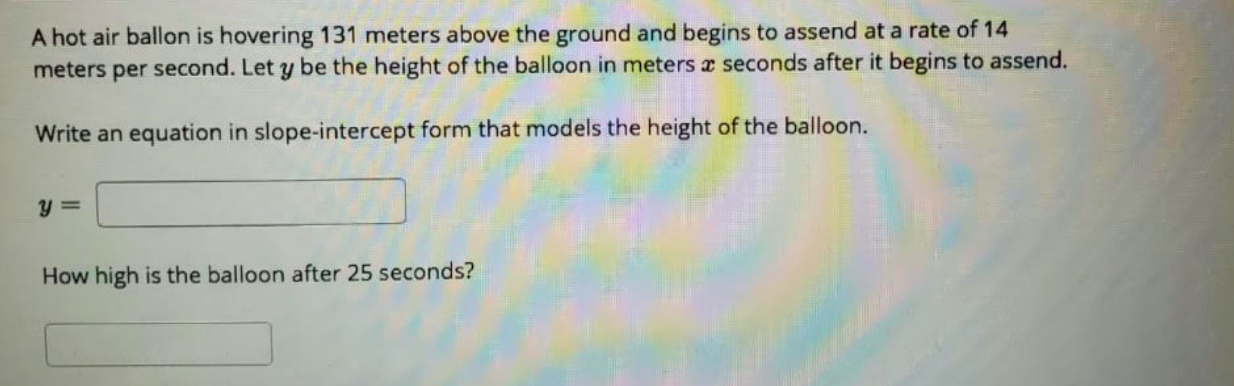# A hot air ballon is hovering 131 meters above the ground and begins to assend at a rate of 14meters per second. Let y be the height of the balloon in meters a seconds after it begins to assend.Write an equation in slope-intercept form that models the height of the balloon.How high is the balloon after 25 seconds?

Question
2 views

this is a precalculus questionhelp_outlineImage TranscriptioncloseA hot air ballon is hovering 131 meters above the ground and begins to assend at a rate of 14 meters per second. Let y be the height of the balloon in meters a seconds after it begins to assend. Write an equation in slope-intercept form that models the height of the balloon. How high is the balloon after 25 seconds? fullscreen
check_circle

Step 1

The initial height of the balloon is 131 meters.

The balloon begins to ascend at a rate of 14 meters per second.

So after x seconds, it wil...

### Want to see the full answer?

See Solution

#### Want to see this answer and more?

Solutions are written by subject experts who are available 24/7. Questions are typically answered within 1 hour.*

See Solution
*Response times may vary by subject and question.
Tagged in